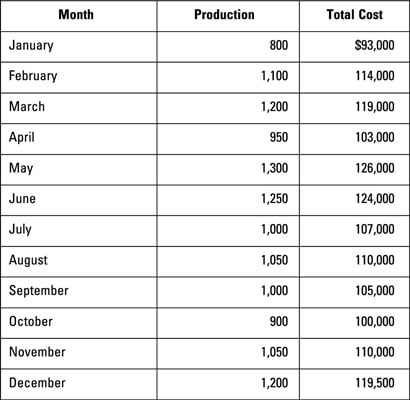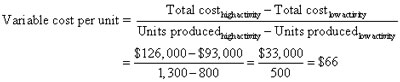##### Managerial Accounting For DummiesThe high-low method enables you to estimate variable and fixed costs based on the highest and lowest levels of activity during the period. Just follow three steps:

1. Based on a table of total costs and activity levels, determine the high and low activity levels.Look at the production level and total costs to identify the high and low activity levels. Xeon Company’s highest production level occurred in May, when the company produced 1,300 units at a total cost of \$126,000. The lowest production level occurred in January, when the company produced just 800 units costing \$93,000.

2. Use the high and low activity levels to compute the variable cost
per unit.

To figure out the variable cost per unit, divide the change in total cost by the change in activity:3. Figure out the total fixed cost.

To compute the total cost, pick either the high or the low cost information (either one works). Plug this information, along with the variable cost per unit from the preceding section, into the total cost formula.

Based on your answer, you can determine that making 1,000 units would mean total variable costs of \$66,000 (1,000 units x \$66 per unit). Total fixed costs equal \$40,200. Therefore, total costs would equal \$106,200:

Total cost = (Variable cost per unit x Units produced) + Total fixed cost
Total cost = (\$66 x 1,000 units) + \$40,200 = \$106,200

The high-low method focuses only on two points: the highest and lowest activity levels. When using this method, don’t get confused by activity levels between these two points, even if their costs are out of the bounds of the costs of the highest and lowest activity levels.